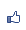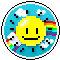唐老鴨之家 市長：燢 　副市長： 加入本城市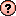｜推薦本城市｜加入我的最愛｜訂閱最新文章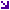本城市首頁討論區精華區投票區影像館推薦連結公告區訪客簿市政中心 (0)益智問題求解之一 瀏覽700｜回應0｜推薦0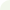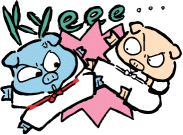燢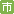等級：6 留言｜加入好友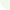□□
╳　□
────
□□
＋□□
────
□□

□中填入1,2,3,...,9，不可重複，可知答案為何？ (17, 4, 68, 25, 93為唯一解)

Dim selected(10) As Boolean
Private Sub CommandButton1_Click()
For X = 12 To 98
For Y = 2 To 9
For Z = 12 To 98
For i = 1 To 9
selected(i) = False
Next i
If (check(Int(X / 10))) Then GoTo nnext
If (check(X Mod 10)) Then GoTo nnext
If (check(Y)) Then GoTo nnext
t1 = X * Y
If (t1 > 99) Then GoTo nnext
If (check(Int(t1 / 10))) Then GoTo nnext
If (check(t1 Mod 10)) Then GoTo nnext
If (check(Int(Z / 10))) Then GoTo nnext
If (check(Z Mod 10)) Then GoTo nnext
t2 = t1 + Z
If (t2 > 99) Then GoTo nnext
If (check(Int(t2 / 10))) Then GoTo nnext
If (check(t2 Mod 10)) Then GoTo nnext
Cells(1, 1) = X
Cells(2, 1) = Y
Cells(3, 1) = X * Y
Cells(4, 1) = Z
Cells(5, 1) = X * Y + Z
nnext:
Next Z
Next Y
Next X
End Sub

Function check(numb)
If (selected(numb)) Then
check = True
Else
selected(numb) = True
check = False
End If
End Function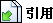引用網址：https://city.udn.com/forum/trackback.jsp?no=58536&aid=5469269